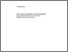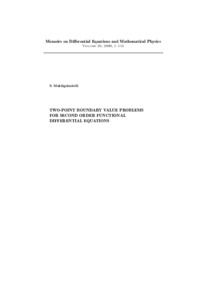Two-point boundary value problems for second order functional differential equations.

Mukhigulashvili, Sulkhan (2000) Two-point boundary value problems for second order functional differential equations. Other. Memoirs on Differential equations and Mathematical Physics.Preview
Text
1-monohraph-memuars.pdfView Item